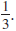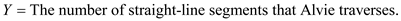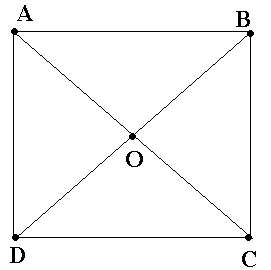Problem

# Alvie Singer lives at 0 in the accompanying diagram and has four friends who live at A,...

Alvie Singer lives at 0 in the accompanying diagram and has four friends who live at A, B, C, and D. One day Alvie decides to go visiting, so he tosses a fair coin twice to decide which of the four to visit. Once at a friend’s house, he will either return home or else proceed to one of the two adjacent houses (such as 0, A, or C when at B), with each of the three possibilities having probability . In this way, Alvie continues to visit friends until he returns home.a. Let X = the number of times that Alvie visits a friend. Derive the pmf of X.

b. Let Y= the number of straight-line segments that Alvie traverses (including those leading to and from 0). What is the pmf of Y?

c. Suppose that female friends live at A and C and male friends at B and D. If Z = the number f visits to female friends, what is the pmf of Z?

#### Step-by-Step Solution

Solution 1

a) Alvie chooses the four friends to be visited, each with equal probability

Once at a friend's house, Alvie will either return home or else proceed to one of the two adjacent houses.

That is if he is at $$B$$, he proceeds to $$\mathrm{O}$$ or $$\mathrm{A}$$ or $$\mathrm{C}$$

Probability of each of these three events is $$\frac{1}{3}$$

$$X$$ is defined as the number of times that Alvie visits a friend

The possible values that $$X$$ can take are $$\{1,2,3,4, \ldots . .\}$$

$$X$$ takes value 1 if Alvie visits a friend once

This happens when Alvie returns home after visiting a friend

The probability that he returns home from a friend's house $$=\frac{1}{3}$$

So we have $$P(X=1)=\frac{1}{3}$$

| x | 1 | 2 | 3 | dots dots dots. | x | |

| :--- | :---: | :---: | :---: | :---: | :---: | :---: |

| p(x) | (1)/(3) | (2)/(9) | (4)/(27) | dots dots dots.. | ((2)/(3))^(x-1)*(1)/(3) | dots dots dots.. |

(b) The random variable Y is defined as:`If Alvie visits a friend once he has to traverse through two line segments

For example if he visits only $$B$$, he has to traverse through the line segment $$O B$$ and then again as he has to go home immediately after this visit, he has to traverse through the line segment BO.

So if Alvie visits a friend once, the number of line segments that he has to traverse $$=2$$

Probability that he traverses 2 line segments is therefore equal to the probability that the number of times he visits a friend is 1

In other words,

\begin{aligned} P(Y=2) &=P(X=1) \\ &=\frac{1}{3} \end{aligned}

If the number of times that Alive visits a friend is 2 , he has to traverse through three line segments

For example if he visits only $$B$$, and then he visits $$A$$, he has to traverse through the line segment $$O B$$ and then $$B A$$, and again as he has to go home immediately after this visit, he has to traverse through the line segment $$A O$$.

So if the number of times that Alive visits a friend is 2 , the number of line segments that he has to traverse $$=3$$

Probability that he traverses 3 line segments is therefore equal to the probability that the number of times he visits a friend is 2

In other words,

\begin{aligned} P(Y=3) &=P(X=2) \\ &=\frac{1}{3}\left(\frac{2}{3}\right) \end{aligned}

| y | 2 | 3 | dots dots dots. | y |

| :--- | :--- | :--- | :--- | :--- |

| p(y) | (2)/(9) | (4)/(27) | dots dots dots dots+dots dots | |

| ((2)/(3))^(y-2) | (1)/(3) | dots dots dots. | | |

Since the visits are independent, we get:

\begin{aligned} p(1) &=\left(\frac{1}{2} \times \frac{1}{3}\right)+\left(\frac{1}{2} \times \frac{2}{3} \times \frac{1}{3}\right)+\left(\frac{1}{2} \times \frac{2}{3} \times \frac{1}{3}\right)+\left(\frac{1}{2} \times \frac{2}{3} \times \frac{2}{3} \times \frac{1}{3}\right) \\ &=\left(\frac{1}{2} \times \frac{1}{3}\right)\left(1+\frac{2}{3}\right)+\left(\frac{1}{2} \times \frac{2}{3} \times \frac{1}{3}\right)\left(1+\frac{2}{3}\right) \\ &=\left(\frac{1}{2}\right)\left(1+\frac{2}{3}\right)\left(\frac{1}{3}\right)+\left(\frac{1}{2} \times \frac{2}{3}\right)\left(1+\frac{2}{3}\right)\left(\frac{1}{3}\right) \\ &=\left(\frac{1}{2}\right)\left(\frac{5}{3}\right)\left(\frac{1}{3}\right)+\left(\frac{1}{2}\right)\left(\frac{2}{3}\right)\left(\frac{5}{3}\right)\left(\frac{1}{3}\right) \end{aligned}

The first term in the above expression corresponds to the probability of first visiting a female friend and the second term corresponds to the probability of first visiting a male friend.

So proceeding in the similar way, we can write:

\begin{aligned} p(2) &=\left(\frac{1}{2}\right)\left(\frac{2}{3}\right)^{2}\left(\frac{5}{3}\right)\left(\frac{1}{3}\right)+\left(\frac{1}{2}\right)\left(\frac{2}{3}\right)^{3}\left(\frac{5}{3}\right)\left(\frac{1}{3}\right) \\ &=\left(\frac{1}{2}\right)\left(\frac{2}{3}\right)^{2}\left(\frac{5}{3}\right)\left(\frac{1}{3}\right)+\left(\frac{1}{2}\right)\left(\frac{2}{3}\right)^{2}\left(\frac{2}{3}\right)\left(\frac{5}{3}\right)\left(\frac{1}{3}\right) \\ p(3) &=\left(\frac{1}{2}\right)\left(\frac{2}{3}\right)^{4}\left(\frac{5}{3}\right)\left(\frac{1}{3}\right)+\left(\frac{1}{2}\right)\left(\frac{2}{3}\right)^{4}\left(\frac{2}{3}\right)\left(\frac{5}{3}\right)\left(\frac{1}{3}\right) \\ \text { Generalizing this series, we get the pmf of } Z \text { is obtained as follows: } \\ p(z) &=\left(\frac{1}{2}\right)\left(\frac{2}{3}\right)^{2 z-2}\left(\frac{5}{3}\right)\left(\frac{1}{3}\right)+\left(\frac{1}{2}\right)\left(\frac{2}{3}\right)^{2 z-2}\left(\frac{2}{3}\right)\left(\frac{5}{3}\right)\left(\frac{1}{3}\right) \\ &=\left(\frac{2}{3}\right)^{2 z-2}\left[\left(\frac{1}{2}\right)\left(\frac{5}{3}\right)\left(\frac{1}{3}\right)+\left(\frac{2}{3}\right)\left(\frac{1}{2}\right)\left(\frac{5}{3}\right)\left(\frac{1}{3}\right)\right] \\ &=\left(\frac{2}{3}\right)^{2 z-2}\left[\left(\frac{5}{18}\right)+\left(\frac{10}{54}\right)\right] \\ &=\left(\frac{2}{3}\right)^{2 z-2}\left(\frac{25}{54}\right) \end{aligned}